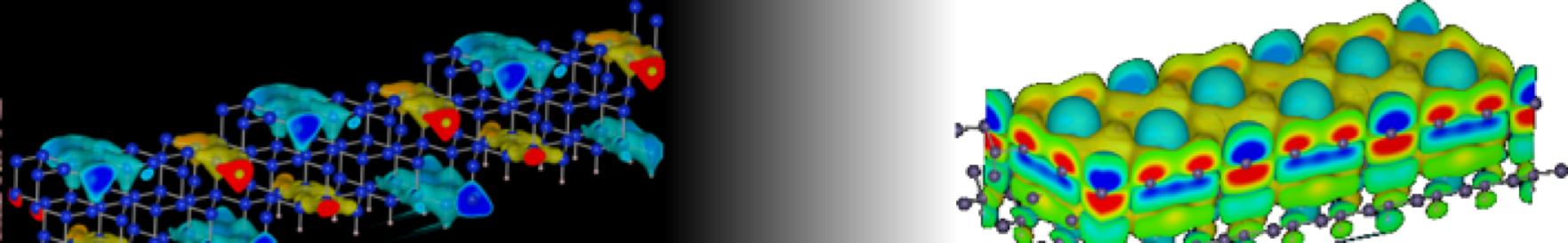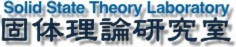SST Lab Dokuwiki Header#@surface

### サイドバー

SST Lab Dokuwiki Header## Contents

### Keep Out

ab_initio:subsetオブジェクト

# Subsetオブジェクト

Using the Subset filter you can make a subset of a listofatoms object. You construct the filter like this:

from ASE.Filters.Subset import Subset
m = Subset(atoms,mask=mask)

… or

m = Subset(atoms,indices=indices)

mask is a list of length N, N being the number of atoms. A zero value for an element in mask signifies that this atoms is not included in the subset.

indices is a list of indices for atoms which should be included in the subset.

It can be usefull if you have many atoms, to use the tag attribute on the individual atoms then constructing the listofatoms object (see atoms).

By setting a non zero tag (ex 117) on all the static atoms, the mask can be constructed like:

mask = [a.GetTag() != 117 for a in atoms]

Example of use (freezing some degrees of freedom):

>>> from ASE import Atom, ListOfAtoms
>>> from ASE.Filters.Subset import Subset
>>> m = ListOfAtoms([Atom('O', (0, 0, 0), tag=117),
...                  Atom('H', (0.773, 0.600, 0)),
...                  Atom('H', (-0.773, 0.600, 0))])
>>> m1 = Subset(m, indices=[1, 2])
>>> m2 = Subset(m, mask=[0, 1, 1])
>>> mask=[a.GetChemicalSymbol() == 'H' for a in m]
[0, 1, 1]
>>> m4 = Subset(m, mask=[a.GetTag() != 117 for a in m])
>>>
>>> m5 = Subset(m, indices=)
>>> m6 = Subset(m, mask=[1, 0, 0])
>>> m7 = Subset(m, mask=[a.GetChemicalSymbol() == 'O' for a in m])
>>> m8 = Subset(m, mask=[a.GetTag() == 117 for a in m])
>>>
>>> m4.GetCartesianPositions()
array([[ 0.773,  0.6  ,  0.   ],
[-0.773,  0.6  ,  0.   ]])
>>> m8.GetCartesianPositions()
array([       [ 0.,  0.,  0.]])

The first four filters are idential - they all behave like ListOfAtoms objects with only two hydrogen atoms. The last four filters all behave like ListOfAtoms objects with only one oxygen atom.

ab_initio/subsetオブジェクト.txt · 最終更新: 2020/08/17 16:01 by kimi

### ページ用ツール